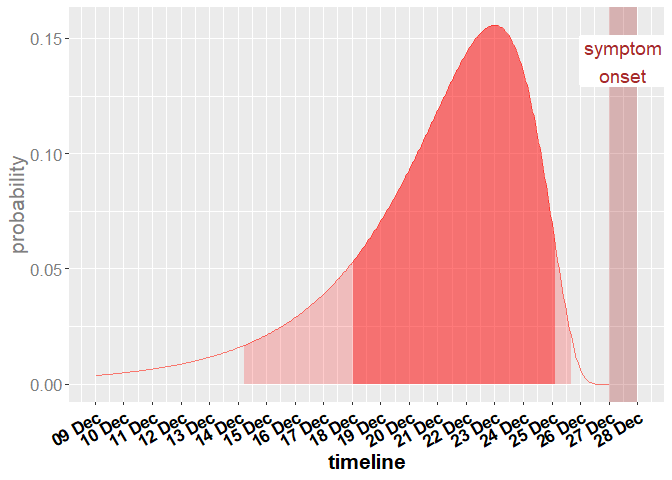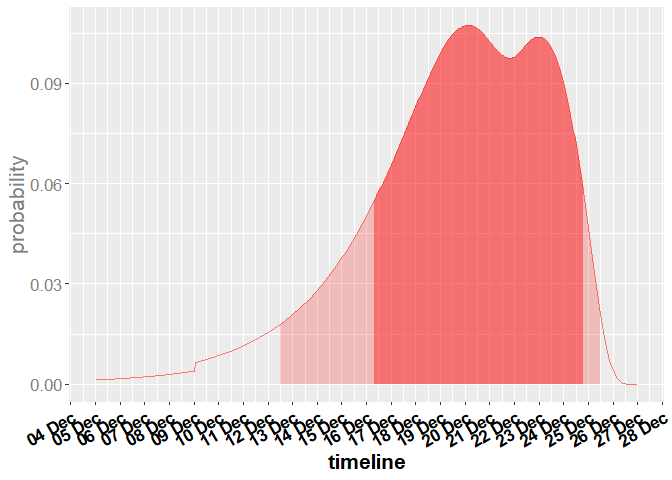# Statistical Modelling for Infectious Disease Management - Infection period

## Question

One or several persons start to show symptoms of COVID-19. When did the person become infected?

## Generating a data frame with dates and infection probability using get_infection_density for one person

The function get_infection_density() can be used to calculate a data frame containing the infection probability when the person shows symptoms.

### Inputs

The function get_infection_density() expects the following input arguments:

First, the symptom_begin_date is needed, which defines when the person started to have symptoms.

Then, the max_incubation_days has to be set, which defines the interval length of the distribution output.

The remaining inputs meanlog and sdlog are the parameters of the log-normal distribution for the infection probability.

symptom_begin_date <- as.Date("2021-12-28")
max_incubation_days <- 18
meanlog <- 1.69
sdlog <- 0.55

infec_date_df <- get_infection_density(symptom_begin_date,
max_incubation_days,
meanlog,
sdlog)

### Methodology

The default values of log-normal distribution are taken from the paper Xin et al . In this paper the authors made a systematic review of the current literature and estimated those parameters based on their meta-analysis.

### Output

The data frame shows for each hour from the earliest potential start of infection up to the symptom begin date the resulting density of the log-normal distribution. This density can be used for calculating the most probable period of the infection.

values 100 to 109 of resulting data frame
dates distribution
100 2021-12-14 04:00:00 0.0122820
101 2021-12-14 05:00:00 0.0124346
102 2021-12-14 06:00:00 0.0125891
103 2021-12-14 07:00:00 0.0127457
104 2021-12-14 08:00:00 0.0129043
105 2021-12-14 09:00:00 0.0130650
106 2021-12-14 10:00:00 0.0132277
107 2021-12-14 11:00:00 0.0133926
108 2021-12-14 12:00:00 0.0135597
109 2021-12-14 13:00:00 0.0137289

## Generating a data frame with dates and probability of infection using get_misc_infection_density for several persons

The function get_misc_infection_density() creates a data frame containing the mixture probability of all considered persons. It can be used to give an overview of the infection probability of several persons with symptom onset dates, e.g., one person with symptom onset on 24.12.2021 and two persons with symptom onset on 28.12.2021.

### Inputs

The following arguments are needed for using the function get_misc_infection_density():

The first parameter symptom_begin_dates contains the dates when the persons got symptoms.

The second parameter persons contains the number of persons having symptoms on each date.

The remaining inputs are the same as in get_infection_density.

symptom_begin_dates <- c(as.Date("2021-12-24"), as.Date("2021-12-28"))
persons <- c(1, 2)
max_incubation_days <- 18

misc_infec_date_df <- get_misc_infection_density(symptom_begin_dates,
persons,
max_incubation_days)

### Methodology

This function uses the get_infection_density function and generates a mixture distribution . This probability distribution is obtained by a sum of the infection probability distribution for each symptom onset day multiplied by the percentage of persons, which have started to show symptoms on this day.

### Output

The data shows the mixture log-normal distribution and thus gives an overview of the potential infection time points for all considered persons. However, it does not necessarily have to imply that they had their infection on the same time point. In fact, there did not have to be an event, where the persons met. It shows when the persons got infected and it is possible that there is more than one infection date, which can be seen based on several maxima.

values 100 to 109 of resulting data frame
dates distribution
100 2021-12-10 04:00:00 0.0066933
101 2021-12-10 05:00:00 0.0067743
102 2021-12-10 06:00:00 0.0068564
103 2021-12-10 07:00:00 0.0069395
104 2021-12-10 08:00:00 0.0070237
105 2021-12-10 09:00:00 0.0071090
106 2021-12-10 10:00:00 0.0071953
107 2021-12-10 11:00:00 0.0072828
108 2021-12-10 12:00:00 0.0073713
109 2021-12-10 13:00:00 0.0074610

## Visualization example of the data frame of get_infection_density

Source
.calculate_qstart_qend <- function(probability, df) {
hdr_df <- hdr(den = data.frame(x = 1:length(df$distribution), y = df$distribution),
p = probability * 100)$hdr qstart <- (hdr_df[1:(length(hdr_df) / 2) * 2] - 1) / 24 qend <- (hdr_df[1:(length(hdr_df) / 2) * 2 - 1] - 1) / 24 return(list("qstart" = qstart, "qend" = qend)) } Source .shade_curve <- function(df, qstart, qend, fill = "red", alpha = 0.4) { subset_df <- df[floor(qstart * 24):ceiling(qend * 24), ] geom_area(data = subset_df, aes(x = x, y = y), fill = fill, color = NA, alpha = alpha) } Source  symptom_begin_date <- as.Date("2021-12-28") df <- infec_date_df period_80 <- .calculate_qstart_qend(0.8, df) period_95 <- .calculate_qstart_qend(0.95, df) symp_date_posixct_start <- as.POSIXct(format(as.POSIXct(symptom_begin_date, tz = "CET"), "%Y-%m-%d")) symp_date_posixct_end <- as.POSIXct(format(as.POSIXct(symptom_begin_date + 1, tz = "CET"), "%Y-%m-%d")) symp_date_posixct_mid <- symp_date_posixct_start - as.numeric(difftime(symp_date_posixct_start, symp_date_posixct_end, units = "hours")) / 2 * 3600  Source  g <- ggplot() + scale_x_datetime(breaks = scales::date_breaks("1 days"), labels = scales::date_format("%d %b")) + theme(axis.text.x = element_text(angle = 90)) + # scale_x_continuous(breaks = x_tick, # labels = x_label) + # theme(axis.ticks.x = element_line(color = c(rbind(rep("black", length(x_label) / 2), rep(NA, length(x_label) / 2))), linetype = 2, size = 1))+ geom_path(aes(x = df$dates, y = df$distribution, color = "red")) + .shade_curve(df = data.frame(x = df$dates, y = df$distribution), period_80$qstart,
period_80$qend) + .shade_curve(df = data.frame(x = df$dates, y = df$distribution), period_95$qstart,
period_95$qend, alpha = 0.2) + geom_rect(data = data.frame(xmin = symp_date_posixct_start, xmax = symp_date_posixct_end, ymin = -Inf, ymax = Inf), aes(xmin = xmin, xmax = xmax, ymin = ymin, ymax = ymax), fill = "brown", alpha = 0.3) + geom_label(aes(x = symp_date_posixct_mid, y = 0.9*max(df$distribution), label = "symptom\nonset"),
colour = "brown", fill = "white", size = 5, label.size = NA) +
ylab("probability") +
xlab("timeline") +
labs(color = 'Verteilung') +
# ggtitle("Visualization of get_infection_density ") +
theme(legend.position = "none", text = element_text(size = 16*5/5)) +
theme(axis.text.x = element_text(colour = "black", face = "bold", angle = 30, hjust = 1)) +
theme(axis.title.x = element_text(colour = "black", face = "bold")) +
theme(axis.text.y = element_text(colour = "gray50")) +
theme(axis.title.y = element_text(colour = "gray50"))

g## Visualization example of the data frame of get_misc_infection_density

Source
  df <- misc_infec_date_df

period_80 <- .calculate_qstart_qend(0.8, df)
period_95 <- .calculate_qstart_qend(0.95, df)

symp_date_posixct_start <- as.POSIXct(format(as.POSIXct(symptom_begin_date, tz = "CET"), "%Y-%m-%d"))
symp_date_posixct_end <- as.POSIXct(format(as.POSIXct(symptom_begin_date + 1, tz = "CET"), "%Y-%m-%d"))
symp_date_posixct_mid <- symp_date_posixct_start - as.numeric(difftime(symp_date_posixct_start,
symp_date_posixct_end, units = "hours")) / 2 * 3600

Source
  g <- ggplot() +

scale_x_datetime(breaks = scales::date_breaks("1 days"), labels = scales::date_format("%d %b")) +
theme(axis.text.x = element_text(angle = 90)) +
# scale_x_continuous(breaks = x_tick,
#                    labels = x_label) +
# theme(axis.ticks.x = element_line(color = c(rbind(rep("black", length(x_label) / 2), rep(NA, length(x_label) / 2))),        linetype = 2, size = 1))+
geom_path(aes(x = df$dates, y = df$distribution, color = "red")) +
.shade_curve(df = data.frame(x = df$dates, y = df$distribution),
period_80$qstart, period_80$qend) +
.shade_curve(df = data.frame(x = df$dates, y = df$distribution),
period_95$qstart, period_95$qend,
alpha = 0.2) +
ylab("probability") +
xlab("timeline") +
labs(color = 'Verteilung') +
# ggtitle("Visualization of get_infection_density") +
theme(legend.position = "none", text = element_text(size = 16 * 5 / 5)) +
theme(axis.text.x = element_text(colour = "black", face = "bold", angle = 30, hjust = 1)) +
theme(axis.title.x = element_text(colour = "black", face = "bold")) +
theme(axis.text.y = element_text(colour = "gray50")) +
theme(axis.title.y = element_text(colour = "gray50"))

g## Literature

 Xin H, Wong JY, Murphy C, Yeung A, Taslim Ali S, Wu P, Cowling BJ. The Incubation Period Distribution of Coronavirus Disease 2019: A Systematic Review and Meta-Analysis. Clinical Infectious Diseases, 2021; 73(12): 2344-2352.